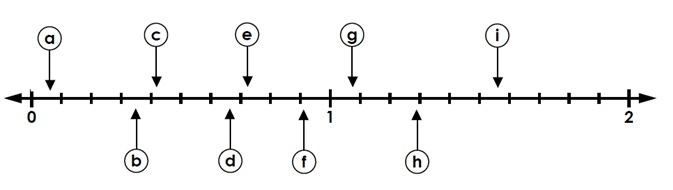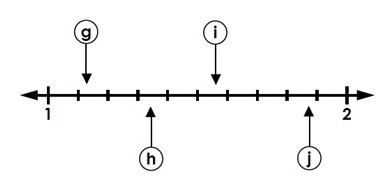# Reading Decimal Position on a Number Line: Hundredths

#### Complete Python Prime Pack

9 Courses     2 eBooks

#### Artificial Intelligence & Machine Learning Prime Pack

6 Courses     1 eBooks

#### Java Prime Pack

9 Courses     2 eBooks

Consider the number line given belowIt is divided into two major divisions 0-1 and 1-2. Each of this is further divided into 10 divisions which are tenths. The numbers in between the tenths are the hundredths. The letters shown on the number line represent hundredths.

Consider reading the decimal 0.35 represented by letter b on the number line. It is thirty-five hundredths and is less than one. so it lies between 0 and 1; more precisely midway between thirty hundredths (three tenths) and forty hundredths (four tenths)

So we read the decimal 0.35 or thirty-five hundredths on the number line as a point midway between 0.30 and 0.40 or 0.3 and 0.4

Similarly, a represents 0.06; c is 0.42; d is 0.67; e is 0.72; f is 0.91; g, h and i represent values greater than 1. g is 1.08; h is 1.29; and i is 1.57

Identify the decimal numbers denoted by the letters on the number line given below.### Solution

Step 1:

a represents a value lying between 0 and 0.1. It represents 6 hundredths or 0.06.

Step 2:

Similarly, b is 0.27; c is 0.43; d is 0.67; e is 0.92

Identify the decimal numbers denoted by the letters on the number line given below.### Solution

Step 1:

g represents a value lying between 1.1 and 1.2 It represents 12 hundredths or 1.12.

So g represents 1.12;

Step 2:

Similarly, h is 1.34; i is 1.58 and j is 1.88